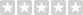Latest

# TEA1101P

Product Code: Charger
Availability: 10
Price: 7,000Riel.‌‌‌រ
Qty:     - OR -0 reviews  |  Write a review

CONTROL FOR BATT.CHARGER,PHILIPS

 Documentation DatasheetProduct Specification

 Feature Battery Charger 4.1 Battery Charger Battery charging is done with the IC TEA1101, TEA1102 and Philips. Since the entire system is only one common mass, the standard circuit was slightly modified. The current sensor is determined by the ground path to the required voltage and Akkuweg fed back through a Differnzverstärker the IC. The whole has the additional advantage that the ADC, the signal the same as the charging current. In order to avoid unnecessary load on the power source, the charging current to 500 mA is limited, as a quick charge at a long-term operation does not make much sense anyway. The trickle charge consists of a 40 ms current pulse widths of 180 mA all 860 ms.Figure 4-2 Since a full charge takes approximately 10 hours, a small heatsink must be donated for T1. The charging current determining resistor R1. For higher charge currents it is reduced and enlarged for larger charging currents. The TEA1101 has a maximum operating voltage of 10.5 volts. However, since the supply voltage is 12 volts, a voltage regulator has to be used. In the circuit of WUDU1 for a 78L08 was used. During the operation, however, he is gone 3 times in the semiconductor sky. Therefore here a stabilization in accordance with the conventional principle of a Zener diode and an NPN transistor is used. The Zener voltage is defined at a current of 5 mA. The controller is operated here by R3 with 4mA, so the setting is a Zener voltage of approximately 8.7 volts. Minus the CE voltage of T3 is then given a stabilized voltage from 8 to 8.1 volts. IC2a the differential amplifier to the load current measurement. He has a gain of 1 and therefore provides an output voltage of Ua = Ilade * R10. Ua [V] = I [A] * R10 = 0.5 A * 0.22 ohms = 0.11 volts. To adjust the tension on the dynamic range of the ADC (4.096 V) in the signal IC2b is enhanced by a factor of 16. Ua = 0.11 * 16 = 1.76 volts. Something safety was left for the event to possibly still to increase the boost power slightly. Its boundary can then lie at 1.16 A. If more charge current is required, the gain must be lowered by reducing the size of R18. The maximum charging voltage is about 9.5 volts. In order to measure the battery voltage is decoupled by a voltage divider sent (R11/R12) and by IC2c. The circuit is calculated, which is generated for the maintenance charge every 910ms, a charge pulse of 42ms. This corresponds to a duty cycle of 4.4%, which is a trickle charge current of about 22mA.

## Write a review